This book is archived and will be removed July 6, 2022. Please use the updated version.

Diffraction

# 25 Single-Slit Diffraction

### Learning Objectives

By the end of this section, you will be able to:

• Explain the phenomenon of diffraction and the conditions under which it is observed
• Describe diffraction through a single slit

After passing through a narrow aperture (opening), a wave propagating in a specific direction tends to spread out. For example, sound waves that enter a room through an open door can be heard even if the listener is in a part of the room where the geometry of ray propagation dictates that there should only be silence. Similarly, ocean waves passing through an opening in a breakwater can spread throughout the bay inside. ((Figure)). The spreading and bending of sound and ocean waves are two examples of diffraction, which is the bending of a wave around the edges of an opening or an obstacle—a phenomenon exhibited by all types of waves.

Because of the diffraction of waves, ocean waves entering through an opening in a breakwater can spread throughout the bay. (credit: modification of map data from Google Earth)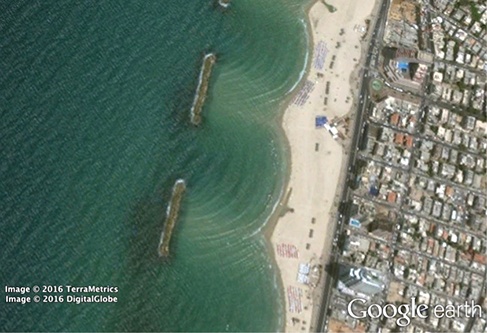The diffraction of sound waves is apparent to us because wavelengths in the audible region are approximately the same size as the objects they encounter, a condition that must be satisfied if diffraction effects are to be observed easily. Since the wavelengths of visible light range from approximately 390 to 770 nm, most objects do not diffract light significantly. However, situations do occur in which apertures are small enough that the diffraction of light is observable. For example, if you place your middle and index fingers close together and look through the opening at a light bulb, you can see a rather clear diffraction pattern, consisting of light and dark lines running parallel to your fingers.

### Diffraction through a Single Slit

Light passing through a single slit forms a diffraction pattern somewhat different from those formed by double slits or diffraction gratings, which we discussed in the chapter on interference. (Figure) shows a single-slit diffraction pattern. Note that the central maximum is larger than maxima on either side and that the intensity decreases rapidly on either side. In contrast, a diffraction grating (Diffraction Gratings) produces evenly spaced lines that dim slowly on either side of the center.

Single-slit diffraction pattern. (a) Monochromatic light passing through a single slit has a central maximum and many smaller and dimmer maxima on either side. The central maximum is six times higher than shown. (b) The diagram shows the bright central maximum, and the dimmer and thinner maxima on either side.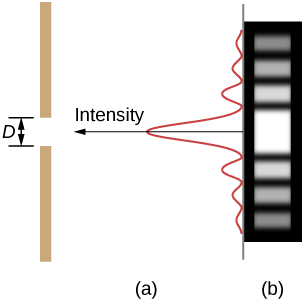The analysis of single-slit diffraction is illustrated in (Figure). Here, the light arrives at the slit, illuminating it uniformly and is in phase across its width. We then consider light propagating onwards from different parts of the same slit. According to Huygens’s principle, every part of the wave front in the slit emits wavelets, as we discussed in The Nature of Light. These are like rays that start out in phase and head in all directions. (Each ray is perpendicular to the wave front of a wavelet.) Assuming the screen is very far away compared with the size of the slit, rays heading toward a common destination are nearly parallel. When they travel straight ahead, as in part (a) of the figure, they remain in phase, and we observe a central maximum. However, when rays travel at an angle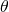relative to the original direction of the beam, each ray travels a different distance to a common location, and they can arrive in or out of phase. In part (b), the ray from the bottom travels a distance of one wavelength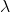farther than the ray from the top. Thus, a ray from the center travels a distance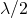less than the one at the bottom edge of the slit, arrives out of phase, and interferes destructively. A ray from slightly above the center and one from slightly above the bottom also cancel one another. In fact, each ray from the slit interferes destructively with another ray. In other words, a pair-wise cancellation of all rays results in a dark minimum in intensity at this angle. By symmetry, another minimum occurs at the same angle to the right of the incident direction (toward the bottom of the figure) of the light.

Light passing through a single slit is diffracted in all directions and may interfere constructively or destructively, depending on the angle. The difference in path length for rays from either side of the slit is seen to be D sin.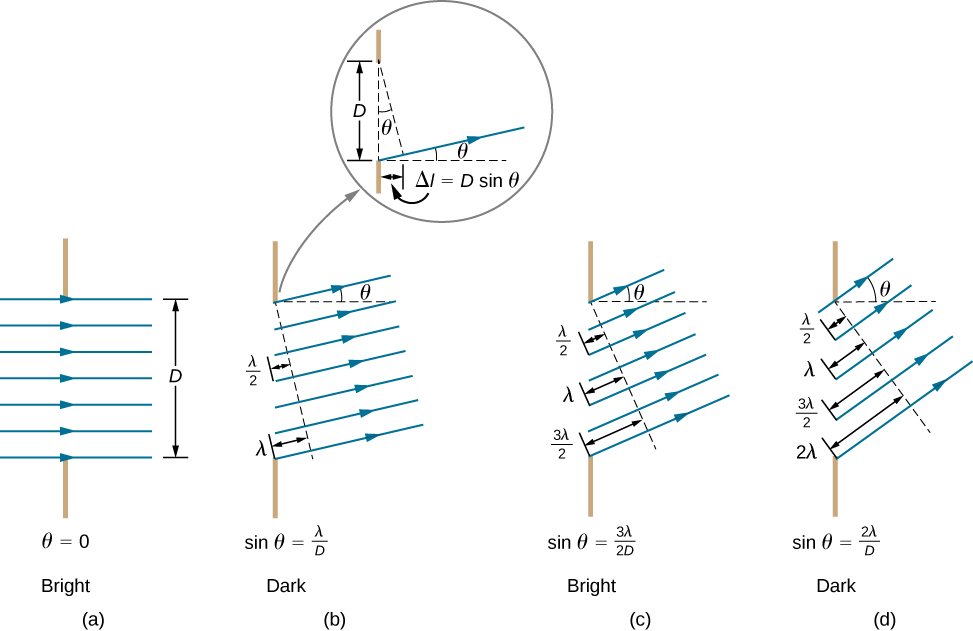At the larger angle shown in part (c), the path lengths differ by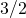for rays from the top and bottom of the slit. One ray travels a distancedifferent from the ray from the bottom and arrives in phase, interfering constructively. Two rays, each from slightly above those two, also add constructively. Most rays from the slit have another ray to interfere with constructively, and a maximum in intensity occurs at this angle. However, not all rays interfere constructively for this situation, so the maximum is not as intense as the central maximum. Finally, in part (d), the angle shown is large enough to produce a second minimum. As seen in the figure, the difference in path length for rays from either side of the slit is D sin, and we see that a destructive minimum is obtained when this distance is an integral multiple of the wavelength.

Thus, to obtain destructive interference for a single slit,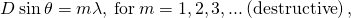where D is the slit width,is the light’s wavelength,is the angle relative to the original direction of the light, and m is the order of the minimum. (Figure) shows a graph of intensity for single-slit interference, and it is apparent that the maxima on either side of the central maximum are much less intense and not as wide. This effect is explored in Double-Slit Diffraction.

A graph of single-slit diffraction intensity showing the central maximum to be wider and much more intense than those to the sides. In fact, the central maximum is six times higher than shown here.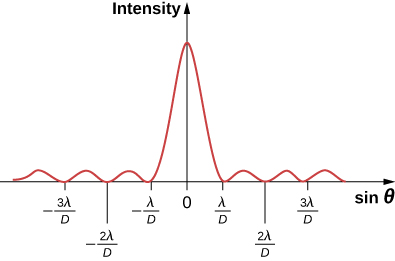Calculating Single-Slit Diffraction Visible light of wavelength 550 nm falls on a single slit and produces its second diffraction minimum at an angle of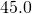relative to the incident direction of the light, as in (Figure). (a) What is the width of the slit? (b) At what angle is the first minimum produced?

In this example, we analyze a graph of the single-slit diffraction pattern.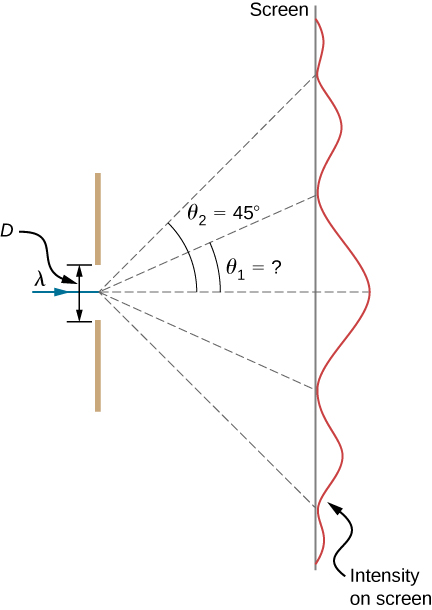Strategy From the given information, and assuming the screen is far away from the slit, we can use the equation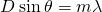first to find D, and again to find the angle for the first minimum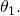Solution

1. We are given that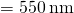,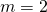, and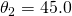. Solving the equationfor D and substituting known values gives2. Solving the equationfor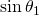and substituting the known values gives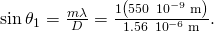Thus the angle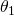is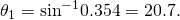Significance We see that the slit is narrow (it is only a few times greater than the wavelength of light). This is consistent with the fact that light must interact with an object comparable in size to its wavelength in order to exhibit significant wave effects such as this single-slit diffraction pattern. We also see that the central maximum extends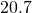on either side of the original beam, for a width of about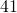. The angle between the first and second minima is only about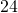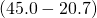. Thus, the second maximum is only about half as wide as the central maximum.

Check Your Understanding Suppose the slit width in (Figure) is increased to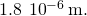What are the new angular positions for the first, second, and third minima? Would a fourth minimum exist?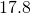,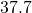,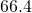; no

### Summary

• Diffraction can send a wave around the edges of an opening or other obstacle.
• A single slit produces an interference pattern characterized by a broad central maximum with narrower and dimmer maxima to the sides.

### Conceptual Questions

As the width of the slit producing a single-slit diffraction pattern is reduced, how will the diffraction pattern produced change?

The diffraction pattern becomes wider.

Compare interference and diffraction.

If you and a friend are on opposite sides of a hill, you can communicate with walkie-talkies but not with flashlights. Explain.

Walkie-talkies use radio waves whose wavelengths are comparable to the size of the hill and are thus able to diffract around the hill. Visible wavelengths of the flashlight travel as rays at this size scale.

What happens to the diffraction pattern of a single slit when the entire optical apparatus is immersed in water?

In our study of diffraction by a single slit, we assume that the length of the slit is much larger than the width. What happens to the diffraction pattern if these two dimensions were comparable?

The diffraction pattern becomes two-dimensional, with main fringes, which are now spots, running in perpendicular directions and fainter spots in intermediate directions.

A rectangular slit is twice as wide as it is high. Is the central diffraction peak wider in the vertical direction or in the horizontal direction?

### Problems

(a) At what angle is the first minimum for 550-nm light falling on a single slit of width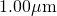? (b) Will there be a second minimum?

a.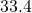; b. no

(a) Calculate the angle at which a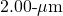-wide slit produces its first minimum for 410-nm violet light. (b) Where is the first minimum for 700-nm red light?

(a) How wide is a single slit that produces its first minimum for 633-nm light at an angle of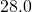? (b) At what angle will the second minimum be?

a.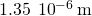; b.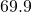(a) What is the width of a single slit that produces its first minimum at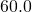for 600-nm light? (b) Find the wavelength of light that has its first minimum at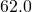.

Find the wavelength of light that has its third minimum at an angle of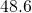when it falls on a single slit of width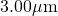.

750 nm

(a) Sodium vapor light averaging 589 nm in wavelength falls on a single slit of width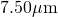. At what angle does it produces its second minimum? (b) What is the highest-order minimum produced?

Consider a single-slit diffraction pattern for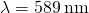, projected on a screen that is 1.00 m from a slit of width 0.25 mm. How far from the center of the pattern are the centers of the first and second dark fringes?

2.4 mm, 4.7 mm

(a) Find the angle between the first minima for the two sodium vapor lines, which have wavelengths of 589.1 and 589.6 nm, when they fall upon a single slit of width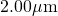. (b) What is the distance between these minima if the diffraction pattern falls on a screen 1.00 m from the slit? (c) Discuss the ease or difficulty of measuring such a distance.

(a) What is the minimum width of a single slit (in multiples of) that will produce a first minimum for a wavelength? (b) What is its minimum width if it produces 50 minima? (c) 1000 minima?

a.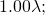b.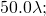c.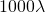(a) If a single slit produces a first minimum at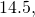at what angle is the second-order minimum? (b) What is the angle of the third-order minimum? (c) Is there a fourth-order minimum? (d) Use your answers to illustrate how the angular width of the central maximum is about twice the angular width of the next maximum (which is the angle between the first and second minima).

If the separation between the first and the second minima of a single-slit diffraction pattern is 6.0 mm, what is the distance between the screen and the slit? The light wavelength is 500 nm and the slit width is 0.16 mm.

1.92 m

A water break at the entrance to a harbor consists of a rock barrier with a 50.0-m-wide opening. Ocean waves of 20.0-m wavelength approach the opening straight on. At what angles to the incident direction are the boats inside the harbor most protected against wave action?

An aircraft maintenance technician walks past a tall hangar door that acts like a single slit for sound entering the hangar. Outside the door, on a line perpendicular to the opening in the door, a jet engine makes a 600-Hz sound. At what angle with the door will the technician observe the first minimum in sound intensity if the vertical opening is 0.800 m wide and the speed of sound is 340 m/s?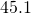### Glossary

destructive interference for a single slit
occurs when the width of the slit is comparable to the wavelength of light illuminating it
diffraction
bending of a wave around the edges of an opening or an obstacle